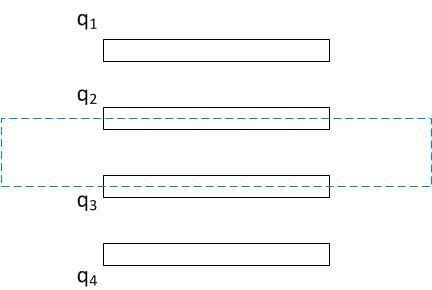# Electrostatics - System of parallel plates

For a system of 'n' parallel plates(metal plates) bearing charges q1, q2,...... qn ; why it is so that the facing surfaces of the plates bear equal and opposite charges ?

How do we prove that ?

BvU
Homework Helper
Gauss' law is very useful for that purpose. Are you familiar with that law already, or do you need an answer without making use of Gauss'law ?

yes I know Gauss' Law

but can we prove it

cnh1995
Homework Helper
Gold Member
yes I know Gauss' Law

but can we prove it
If you have two parallel plates A and B and you put a charge of +10μC on A, it will be distributed on A as +5μC on one surface and +5μC on the other(to make the electric field inside plate A zero). The surface of plate B facing the surface of plate A will have an induced charge of -5μC(and the other surface of plate B will have charge +5μC). This charge is induced in order to terminate the electric field lines from plate A and make the electric field inside plate B zero.

If you have two parallel plates A and B and you put a charge of +10μC on A, it will be distributed on A as +5μC on one surface and +5μC on the other(to make the electric field inside plate A zero). The surface of plate B facing the surface of plate A will have an induced charge of -5μC(and the other surface of plate B will have charge +5μC). This charge is induced in order to terminate the electric field lines from plate A and make the electric field inside plate B zero.

general proof ?

cnh1995
Homework Helper
Gold Member
general proof ?
Basically, charges are induced on metal plates in order to terminate the external electric field lines and keep electric field inside the plates 0. If the plates are close enough (like in case of capacitors), the charges induced will be "equal and opposite" since the electric field between them is of a constant magnitude σ/2ε.
That's all I can say without jumping into math.

BvU
Homework Helper
yes I know Gauss' Law

but can we prove it
Yes. Give it a shot! Where would you locate the Gaussian surface ?

BvU
Homework Helper
So what would be the flux through the dashed surface ?yes
thank u

BvU
•